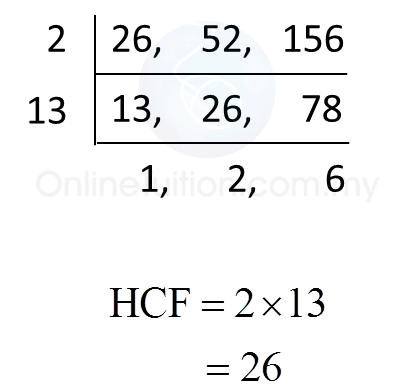# 2.2.3 Factors and Multiples, PT3 Practice

  
             
Question 9:
  Find the lowest common multiple (LCM) of 8, 12 and 15.
  

  Solution:Question 10:
  Find the highest common factor (HCF) of 26, 52 and 156.
  

  Solution:Question 11:
  Given that (m + 5) is the highest common factor of 81 and 108, find the value of m.
  

  Solution: